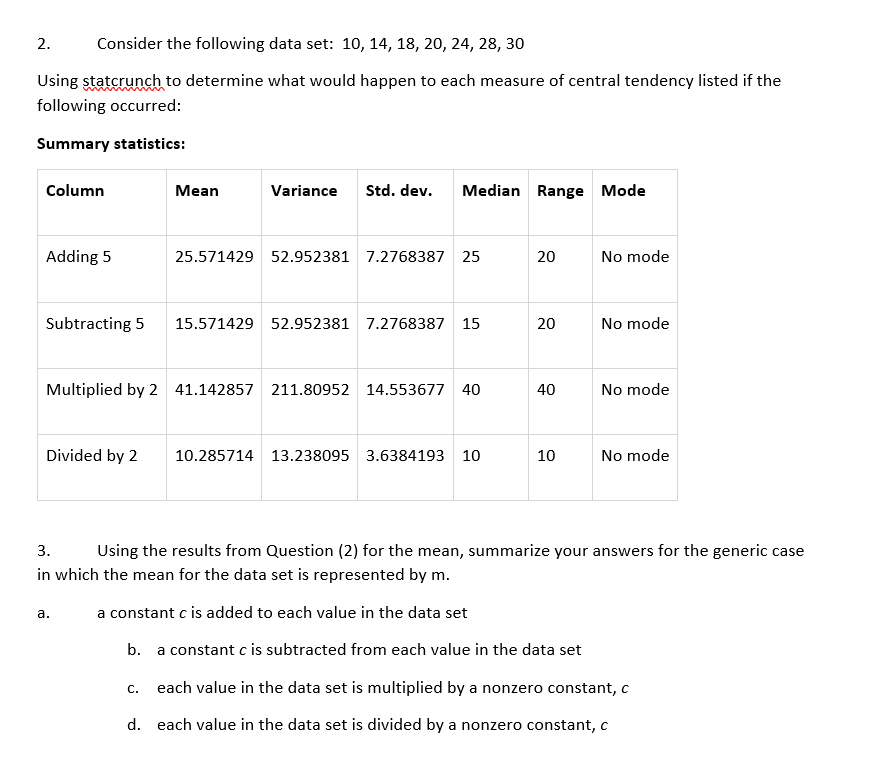# Consider the following data set: 10, 14, 18, 20, 24, 28, 302.Using statcrunch to determine what would happen to each measure of central tendency listed if thefollowing occurred:Summary statisticsStd. devColumnMedian Range ModeМeanVarianceAdding 5No mode25.571429 52.952381 7.2768387 2515.571429 52.952381 7.276838715Subtracting 5No mode20Multiplied by 2 41.142857 211.80952 14.55367740No mode40Divided by 2No mode10.285714 13.238095 3.63841931010Using the results from Question (2) for the mean, summarize your answers for the generic case3.in which the mean for the data set is represented by m.a constant c is added to each value in the data setа.b.a constant c is subtracted from each value in the data seteach value in the data set is multiplied bya nonzero constant, cC.each value in the data set is divided by a nonzero constant, cd.20

Question
2 views

I need assistance with this question number 3. IN the picture attached I provided my results for questions 2.help_outlineImage TranscriptioncloseConsider the following data set: 10, 14, 18, 20, 24, 28, 30 2. Using statcrunch to determine what would happen to each measure of central tendency listed if the following occurred: Summary statistics Std. dev Column Median Range Mode Мean Variance Adding 5 No mode 25.571429 52.952381 7.2768387 25 15.571429 52.952381 7.276838715 Subtracting 5 No mode 20 Multiplied by 2 41.142857 211.80952 14.55367740 No mode 40 Divided by 2 No mode 10.285714 13.238095 3.6384193 10 10 Using the results from Question (2) for the mean, summarize your answers for the generic case 3. in which the mean for the data set is represented by m. a constant c is added to each value in the data set а. b. a constant c is subtracted from each value in the data set each value in the data set is multiplied by a nonzero constant, c C. each value in the data set is divided by a nonzero constant, c d. 20 fullscreen
check_circle

Step 1

3.

It is known that arithmetic mean is dependent of both origin and scale. That is,

Step 2

a).

Therefore, if a constant C is added to each value in the data set, then the resultant mean value will increase by C. That is,

Step 3

b).

If a constant C is subtracted from each value in the data set, then the result...

### Want to see the full answer?

See Solution

#### Want to see this answer and more?

Solutions are written by subject experts who are available 24/7. Questions are typically answered within 1 hour.*

See Solution
*Response times may vary by subject and question.
Tagged in
MathStatistics

### Measures